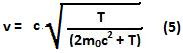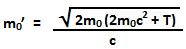## Monday, January 11, 2010

### Irodov Problem 1.385This is a very interesting basic problem that demonstrates how different relativistic collisions are compared to Newtonian collisions - the essential difference being that mass can change in relativity.

After the two masses collide and fuse into a new mass, its rest mass is not simply the sum of rest masses of the two constituent particles but something entirely different. In fact it depends on the energy of the collision!

Throughout the collision two important properties will always hold, i) the momentum will be conserved and ii) the total energy will be conserved. Let the final rest mass of the fused particle be. Let the initial velocity of the left particle be u and let v be the velocity of the combined particle after collision.

Since we know that the kinetic energy of the particle before collision is T we have,Conservation of Momentum
Since there are no external forces on the system, its total momentum must be conserved and so we have,Conservation of Energy

The total energy of the system before collision must be equal to that after collision. The total energy is the sum of the rest mass energies of each of the particles and the kinetic energy of the colliding particle.From Eqn (3) and (4) we obtain,Now we can substitute (5) in (4) to obtain,